Next: 8. Simulation Examples Up: 7.2 Development Previous: 7.2.1 Development Rate Modeling

The development rates usually exhibit a strong variation due to standing wave, scattering and notching effects of the exposing light. Although these inhomogeneities are smoothed by the post-exposure bake the maxima of the development rate can be two orders larger than the minima. This imposes extreme demands onto any surface advancement algorithm. Obviously, the time-steps have to be chosen large enough to pass the rate minima, but at the rate maxima they must be small to prevent an overshooting'' to the next maximum. These requirements are substantially different from an etching simulation since the etch rates are typically more gradual. Nevertheless, all available algorithms are applied to both topographic and lithographic problems. The most prominent and widely used simulation approaches are described below.

String algorithm. One of the first attempts to model the surface advancement goes back to Robert Jewett et al., who proposed the so-called string algorithm in [211,212]. We shall explain the operation principle in two spatial dimensions only. The extension to the third dimension is obvious. The surface is approximated by a series of points joined by straight line segments forming a string. Each point advances along the angle bisector of the two adjoining segments according to the local value of the development or etch rate. During the simulation, the segments are kept roughly equal in length by adding points in regions of expansion of the front, and by deleting points in regions of contraction. Problems are due to loop formation, which especially occurs in case of highly varying spatial rate distributions . Initially small loops develop in the surface, but as the simulation continues, these loops begin to expand and also intersect with each other. The destructive loop behavior is actually caused by the string algorithm itself. During the movement of the segments the normal vectors have to be determined from the local surface. The finite numerical precision results in an inaccurate calculation of the subsequent normal vectors. These errors accumulate and the algorithm fails. To avoid this problem, it is necessary to remove the loops before they become too complex. This delooping'' operation has to be performed every few time-steps . For that purpose the intersection of all the segments in the string must be found so that the loops can be deleted. In two dimension these problems can be solved with moderate computational expense. Although the extension to the third spatial dimension is conceptually obvious, it is far away from just replacing the line segments with a triangular surface mesh. The required operations for a stable performance are quite computation expensive. Instead of cutting lines the intersections of faces have to be calculated, which introduces additional error sources. Strong efforts were made to develop efficient loop detection and removal algorithms [109,214,215,209]. The string algorithm is now claimed to be stable also in three spatial dimensions [110,216,213,217,218]. It is the surface advancement algorithm of the SAMPLE lithography simulator in two as well as three dimensions .

Level set methods. Level set methods have recently been introduced by Stanley Osher and James Sethian . They are based on solving a Hamilton-Jacobi type equation for a level set function. More precisely, the idea is to represent the evolving surface position as the set of all points x such that the level set function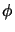(x;t) equals zero. The evolution equation takes the form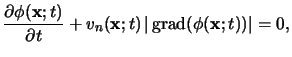(7.26)

whereby vn(x;t) denotes the speed along the surface normal. A convenient initial value condition is given by the distance of every point x to the initial interface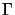(0), i.e.,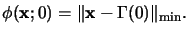(7.27)

(7.28) and (7.29) form an initial value partial differential equation in one dimension higher than the original problem. The propagating surface(t) is obtained from the zero level of the solution(x;t), i.e.,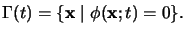(7.28)

The evolution equation (7.28) is the most general form of the level set method. The velocity function vn(x;t) can have arbitrary time-dependence and can change with respect to time. This generality is necessary for advanced etching and deposition simulation since the etch or deposition rates depend on the propagating surface(t) due to shadowing effects. The resulting partial differential equation is then much more involved than it might be expected from (7.28). For lithographic applications two simplifications apply: vn(x;t) is always negative, i.e., only resist removal occurs, and it depends on the precalculated, time-independent development rate. The stationary level set equation takes then the form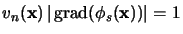(7.29)

and the propagating surface(t) is given by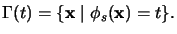(7.30)

The relation (7.31) is the well-known Eikonal equation [11, p. 112].c

Both partial differential equations (7.28) and (7.31) can conveniently be solved with finite-difference methods, whereby care has to be taken to adequately discretize the spatial derivatives in the gradient. A big advantage of the level set approach lies in the fact that the formulation applies to two as well as three spatial dimensions. Generally, the equations can be solved robustly and very fast. For the stationary form (7.31) an acceleration technique called fast marching technique was proposed in . For example, less than one minute simulation time on a SPARC 10 workstation was reported for 200 x 200 x 200 grid points . The various formulations of the level set method have found a wide application for numerous problems in lithography as well as etching and deposition [223,224,225,226]. For example, the lithography simulator DEPICT  and the topography simulator TERRAIN  rely on them. For further reading on level set methods see .d

Cellular algorithms. The third approach was originally proposed by Frederick Dill and relies on a cellular material representation of the underlying geometry . The geometry is divided into a regular array of rectangular prismatic elements. Cells which are exposed constitute the surface. There are two different methods how to propagate the surface, namely the cell-removal algorithm [141,229,213] and the recently proposed structuring element algorithm [230,231,232,233,234]. Cellular algorithms are the most robust of all methods introduced thus far. They are relatively easy to implement in two as well as three spatial dimensions, requiring only a simple set of arrays. We will briefly describe the former algorithm and then discuss the latter one in greater detail since we have adapted it for our development module.

In the cell-removal algorithm the time for the removal of each cell depends on the local development rate and the number of exposed faces. Development proceeds along a surface consisting of unetched cells in contact with etched cells . As the cells are etched away, new cells are exposed to the developer and thus added to the developer. The algorithm, however, is slow and inefficient and can require staggering amounts of computation time and memory in order to produce accurate results. The primary difficulty with the cell-removal algorithm is the trade-off between accuracy and computation time. The accuracy of the simulation increases as the cell-size is decreased, but at the cost of an increased number of cells and an increased computation time. Another serious problem is facet formation. For example, in the case of a uniform isotropic etching simulation beginning from a single seed point the method produces an octagon instead of a circle . This observation led to the development of the previously described string algorithm. Faceting is most probably caused by the use of nearest-neighbor square cells to represent the circular etch. It is extremely troublesome since an increase in cell density decreases the accuracy of the simulation since facets appear more quickly with distance . For lithographic applications this effect is somewhat averaged out and a reasonable accuracy can be achieved . The lithography simulator SOLID  uses this method.

The structuring element algorithm introduced in [230,231] by Ernst Strasser et al. overcomes the shortcomings of the cell-removal algorithm. The algorithm is based on the two fundamental morphological operations of erosion and dilatation. These operations are well-known in digital image processing . The method can be implemented by moving a filter called the structuring element along the surface. Cells within the filter are etched away, while cells outside stay unchanged. A graphical illustration of this operation is shown in Figure 7.3. Within the scope of lithography simulation the structuring element is a circle or sphere in two and three dimensions, respectively, since the development can be modeled as an isotropic etching process . The radius depends on the precalculated development rate multiplied by the chosen time step. The algorithm avoids faceting errors since the filtering implicitly suppresses them at every time step. This is a big advantage of the structuring element algorithm over the cell-removal algorithm. As before, accuracy and run-time depend on the number N of cells. In three dimensions the run-time can be estimated to grow with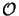(N3), which is two orders more efficient than with the cell removal algorithm . However, a higher cellular resolution is required since the structuring element has to be resolved with sufficient precision.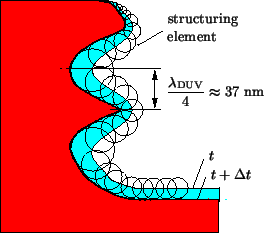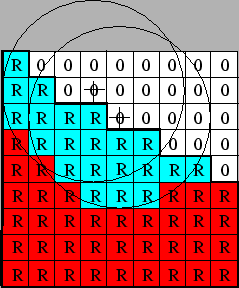For lithographic applications the original proposed structuring element algorithm has to be adapted to account for the strong spatial dependence of the development rate. Possible sources for these variations are standing waves and notching effects occurring during exposure. The required number of cells has to be chosen to resolve them. For example, in the case of standing waves we know that the distance between the maxima and minima of the absorbed light intensity and therefore also of the development rate is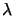/4 yielding approximately 37 nm for DUV illumination at a wavelength=248 nm and a refractive index of 1.65 for the photoresist (c.f. Figure 7.3). For an accurate movement of the development front this significant distance should be resolved by 10 cells. Hence a cell density of 300 cells/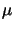m is required. The applicability of the advancement algorithm for this cellular geometry resolution has been demonstrated in . The time-increment is chosen in a way so that the structuring element for the maximal local development rate occurring at a certain time-step comprises roughly 10 cells. Simulation results obtained with the structuring element algorithm are presented in Section 8.2. Exact numbers on run-time and memory demands can also be found there.

#### Footnotes

... p. 112].c
The Eikonal equation forms the foundation of geometrical optics [11, p. 112].
....d
Additional information is available in the WWW at http://math.berkeley.edu/~sethian/level_set.html.Next: 8. Simulation Examples Up: 7.2 Development Previous: 7.2.1 Development Rate Modeling
Heinrich Kirchauer, Institute for Microelectronics, TU Vienna
1998-04-17